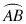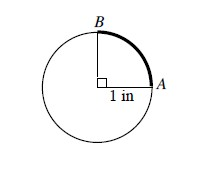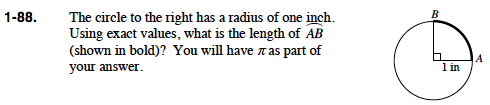### Home > PC > Chapter 1 > Lesson 1.2.2 > Problem1-88

1-88.

The circle below has a radius of one inch. Using exact values, what is the length of(shown in bold)? You will have π as part of your answer. Homework Help ✎What part of the circumference is the arc AB?
How do you know?

$\text{about the whole circle.}$

What is the formula of the circumference of a circle?

$\frac{\pi}{2}$